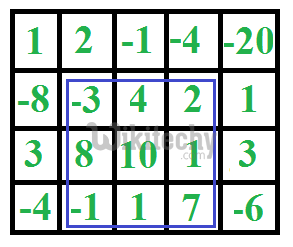# C Programming – Maximum sum rectangle in a 2D matrix

C Programming - Maximum sum rectangle in a 2D matrix - Dynamic Programming Given a 2D array, find the maximum sum subarray in it.For example,

Given a 2D array, find the maximum sum subarray in it. For example, in the following 2D array, the maximum sum subarray is highlighted with blue rectangle and sum of this subarray is 29.This problem is mainly an extension of Largest Sum Contiguous Subarray for 1D array.

The naive solution for this problem is to check every possible rectangle in given 2D array. This solution requires 4 nested loops and time complexity of this solution would be O(n^4).

Kadane’s algorithm for 1D array can be used to reduce the time complexity to O(n^3). The idea is to fix the left and right columns one by one and find the maximum sum contiguous rows for every left and right column pair. We basically find top and bottom row numbers (which have maximum sum) for every fixed left and right column pair. To find the top and bottom row numbers, calculate sun of elements in every row from left to right and store these sums in an array say temp[]. So temp[i] indicates sum of elements from left to right in row i. If we apply Kadane’s 1D algorithm on temp[], and get the maximum sum subarray of temp, this maximum sum would be the maximum possible sum with left and right as boundary columns. To get the overall maximum sum, we compare this sum with the maximum sum so far.

C
``````// Program to find maximum sum subarray in a given 2D array
#include <stdio.h>
#include <string.h>
#include <limits.h>
#define ROW 4
#define COL 5

// Implementation of Kadane's algorithm for 1D array. The function
// returns the maximum sum and stores starting and ending indexes of the
// maximum sum subarray at addresses pointed by start and finish pointers
// respectively.
int kadane(int* arr, int* start, int* finish, int n)
{
// initialize sum, maxSum and
int sum = 0, maxSum = INT_MIN, i;

// Just some initial value to check for all negative values case
*finish = -1;

// local variable
int local_start = 0;

for (i = 0; i < n; ++i)
{
sum += arr[i];
if (sum < 0)
{
sum = 0;
local_start = i+1;
}
else if (sum > maxSum)
{
maxSum = sum;
*start = local_start;
*finish = i;
}
}

// There is at-least one non-negative number
if (*finish != -1)
return maxSum;

// Special Case: When all numbers in arr[] are negative
maxSum = arr;
*start = *finish = 0;

// Find the maximum element in array
for (i = 1; i < n; i++)
{
if (arr[i] > maxSum)
{
maxSum = arr[i];
*start = *finish = i;
}
}
return maxSum;
}

// The main function that finds maximum sum rectangle in M[][]
void findMaxSum(int M[][COL])
{
// Variables to store the final output
int maxSum = INT_MIN, finalLeft, finalRight, finalTop, finalBottom;

int left, right, i;
int temp[ROW], sum, start, finish;

// Set the left column
for (left = 0; left < COL; ++left)
{
// Initialize all elements of temp as 0
memset(temp, 0, sizeof(temp));

// Set the right column for the left column set by outer loop
for (right = left; right < COL; ++right)
{
// Calculate sum between current left and right for every row 'i'
for (i = 0; i < ROW; ++i)
temp[i] += M[i][right];

// Find the maximum sum subarray in temp[]. The kadane()
// function also sets values of start and finish.  So 'sum' is
// sum of rectangle between (start, left) and (finish, right)
//  which is the maximum sum with boundary columns strictly as
//  left and right.
sum = kadane(temp, &start, &finish, ROW);

// Compare sum with maximum sum so far. If sum is more, then
// update maxSum and other output values
if (sum > maxSum)
{
maxSum = sum;
finalLeft = left;
finalRight = right;
finalTop = start;
finalBottom = finish;
}
}
}

// Print final values
printf("(Top, Left) (%d, %d)\n", finalTop, finalLeft);
printf("(Bottom, Right) (%d, %d)\n", finalBottom, finalRight);
printf("Max sum is: %d\n", maxSum);
}

// Driver program to test above functions
int main()
{
int M[ROW][COL] = {{1, 2, -1, -4, -20},
{-8, -3, 4, 2, 1},
{3, 8, 10, 1, 3},
{-4, -1, 1, 7, -6}
};

findMaxSum(M);

return 0;
}``````

Output :

```(Top, Left) (1, 1)
(Bottom, Right) (3, 3)
Max sum is: 29```

Time Complexity: O(n^3)